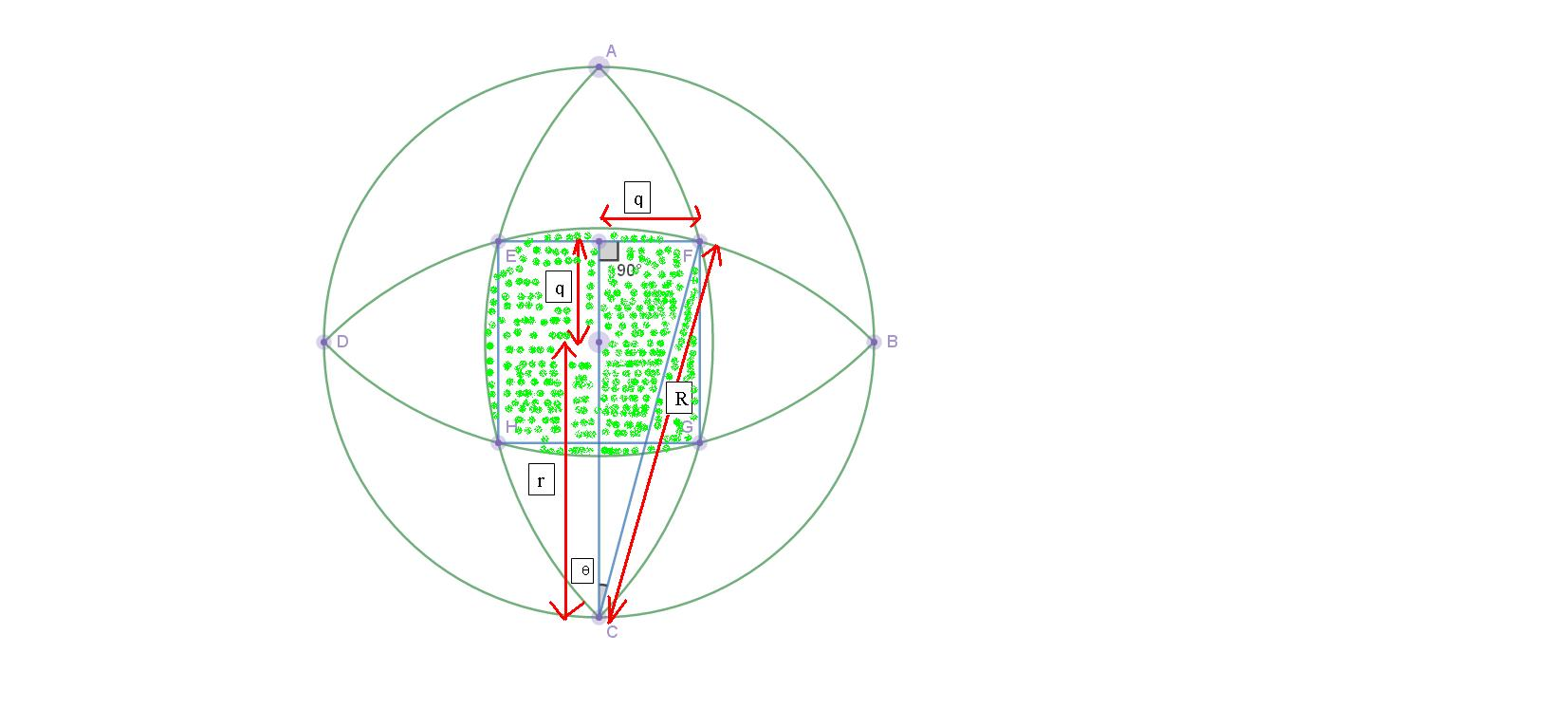# Circles and Arcs$$\text{Diagram shows a circle and } 4 \text{ congruent arcs. The } 4 \text{ arcs are:}$$

$1. \text{ Arc BGHD centered at A}$

$2. \text{ Arc AEHC centered at B }$

$3. \text{ Arc BFED centered at C }$

$4. \text{ Arc AFGC centered at D }$

$\text{ AB, BC, CD, and DA are each }90^\circ \text{arcs}$

$\text{Given:}$

$r = \text{Radius of the circle}$

$R = \text{Radius of each arc}$

$\text{Let EF } = 2q$

$\text{Let } \theta = \angle \text{ACF }$

$\text{Using Pythagorean Theorem}$

$R^2 = r^2 + r^2 \Leftrightarrow R = \sqrt2 r$

$R^2 = q^2 + (q + r)^2 \text{(Using Pythagorean Theorem)}$

$(\sqrt2 r)^2 = q^2 + q^2 + 2qr + r^2$

$2r^2 = 2q^2 + 2qr + r^2$

$2q^2 + 2qr - r^2 = 0$

$q = \dfrac{-2r \pm \sqrt{ (2r)^2 - 4(2)(-r^2) } }{ 2(2) }$

$q = \dfrac{-2r \pm \sqrt{ 12r^2 } }{4}$

$q = \dfrac{-r \pm \sqrt3 (r)}{2}$

$q = \dfrac{ \sqrt3 - 1 }{2} (r) \text{ , } q > 0$

$\sin( \theta ) = \dfrac{q}{R} = \dfrac{ \frac{ \sqrt3 - 1 }{2} (r)}{ \sqrt2 r}$

$\theta = \arcsin\left(\dfrac{ \sqrt3 -1}{2 \sqrt2} \right)$

$\theta = \dfrac{\pi}{12} \text{ rad} = 15^\circ$

$\text{Shaded area} = 4 \times( \text{Area of sector ECF} - \text{Area of} \triangle \text{ECF} ) + \text{Area of square EFGH}$

$\text{Shaded area} = 4 \times\left[ \dfrac{1}{2} \times (\sqrt2 r)^2 (\dfrac{\pi}{12} ) - \dfrac{1}{2} \times (\sqrt2 r)^2 \sin\left( \dfrac{\pi}{12} \right) \right] + \left[2 \times \dfrac{ \sqrt3 - 1 }{2} (r) \right]^2$

$\text{Shaded area} = \dfrac{\pi r^2}{3} - \sqrt2 (\sqrt3 - 1)r ^2 + (\sqrt3 - 1)^2 r^2$

$\text{Shaded area} = \dfrac{\pi r^2}{3} + (\sqrt3 - 1 - \sqrt2 )(\sqrt3 - 1)r ^2$

$\dfrac{ \text{Shaded area} }{ \text{ Total area } } = \dfrac{ \dfrac{\pi r^2}{3} + (\sqrt3 - \sqrt2 - 1 )(\sqrt3 - 1)r ^2 }{\pi r^2}$

$\dfrac{ \text{Shaded area} }{ \text{ Total area } } = \dfrac{ \pi + 3(\sqrt3 - \sqrt2 - 1 )(\sqrt3 - 1) }{3 \pi } = \dfrac{1}{3} + \dfrac{ (\sqrt3 - \sqrt2 - 1 )(\sqrt3 - 1) }{\pi }$Note by Lin Shun Hao
1 year, 3 months ago

This discussion board is a place to discuss our Daily Challenges and the math and science related to those challenges. Explanations are more than just a solution — they should explain the steps and thinking strategies that you used to obtain the solution. Comments should further the discussion of math and science.

When posting on Brilliant:

• Use the emojis to react to an explanation, whether you're congratulating a job well done , or just really confused .
• Ask specific questions about the challenge or the steps in somebody's explanation. Well-posed questions can add a lot to the discussion, but posting "I don't understand!" doesn't help anyone.
• Try to contribute something new to the discussion, whether it is an extension, generalization or other idea related to the challenge.

MarkdownAppears as
*italics* or _italics_ italics
**bold** or __bold__ bold
- bulleted- list
• bulleted
• list
1. numbered2. list
1. numbered
2. list
Note: you must add a full line of space before and after lists for them to show up correctly
paragraph 1paragraph 2

paragraph 1

paragraph 2

[example link](https://brilliant.org)example link
> This is a quote
This is a quote
    # I indented these lines
# 4 spaces, and now they show
# up as a code block.

print "hello world"
# I indented these lines
# 4 spaces, and now they show
# up as a code block.

print "hello world"
MathAppears as
Remember to wrap math in $$ ... $$ or $ ... $ to ensure proper formatting.
2 \times 3 $2 \times 3$
2^{34} $2^{34}$
a_{i-1} $a_{i-1}$
\frac{2}{3} $\frac{2}{3}$
\sqrt{2} $\sqrt{2}$
\sum_{i=1}^3 $\sum_{i=1}^3$
\sin \theta $\sin \theta$
\boxed{123} $\boxed{123}$